Perfect Hollow Boxes

Mike Reid has introduced the concept of Perfect Hollow Boxes (PHBs) which he defines as follows

a perfect hollow box is an a x b x c box with an (a-2) x (b-2) x (c-2) box removed; and also a, b, c >= 3. in other words, it's a 1-unit thick shell of a box.

The diagram below shows boxes for the polycubes up to order 4. These are very easy to form and so no solutions are given.For tricubes a PHB with the requisite volume must be of the form 3p x (3q+2) x r, and for the tetracubes it must be of the form 2p x 2q x r. Both the tricubes above can make all such boxes and certainly the 1x2x2 tetracube can make a 2p x 2q x r box.

We could also look for PHBs in more than three dimensions. Two examples for the tetracubes are shown here.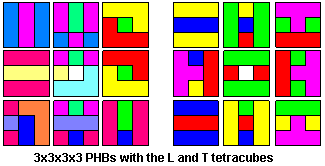Pentacubes

PHB boxes for pentacubes must of one of three forms

 A: 5p x (5q+2) x r B: (5p+1) x (5q+3) x (5r+3) C: (5p+1) x (5q+4) x (5r+4)

I: 3x5x7 (A)

Two symmetric and one asymmetric tilings of the ends gives seven packings. One is shown here to see the full set click on the image.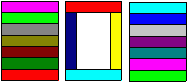P: According to Patrick Hamlyn there are 101 packings of the 3x3x6 and well over 10 000 for the 3x6x8.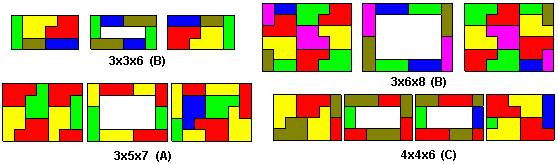Below are two examples of a four dimensional PHB.F: 3x7x20,3x6x8

This information is by Patrick Hamlyn - the 3x7x20 and 3x6x8 (smallest) here both have unique solutions.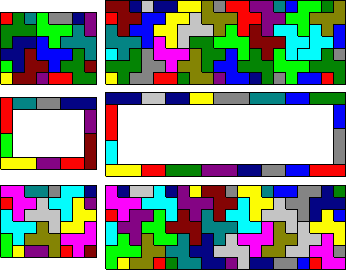5x6x12 has seven solutions. One is shown here to see the full set click on the image.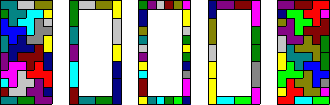N: 4x4x6 - 2 packings, due to a split into two open boxes again (minimal); 4x5x7 - unique; 3x6x8 - five packings.

3x8x11, 4x6x9 (6), 5x5x7 (2)L: Mike Reid found the 4x4x6.

The smallest is the 3x5x7, has 1895 packings.The 4x4x6 has a total of 60 solutions. To see all 60 click on the image below.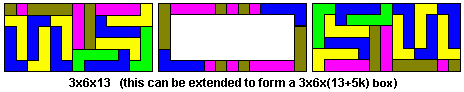V: 4x4x6, 8x8x11

The 4x4x6 is the smallest possible box with just four solutions derived from the diagram belowY: 4x5x12 (56 solutions) , 4x4x16, 4x7x10, 3x7x15 (3 solutions), 3x6x18, 5x5x12, 10x12x16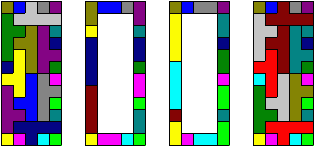U, Z: The smallest box for each is the four dimensional one shown here.This leaves open the cases of the T, W and X pentacubes as well as the three dimensional cases. The X is clearly no possible since it cannot form a corner and almost certainly the majority of the three dimensional pieces cannot form PHBs although at least two can as shown here (the first by Roel Huisman and the second by Mike Reid).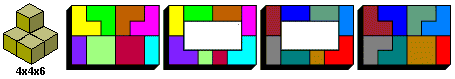Hexacubes

PHB boxes for hexacubes must of one of three forms

 A: 3p x (3q+2) x r B: (6p+1) x (6q+3) x (3r+2) C: (6p+2) x (6q+2) x (6r+3)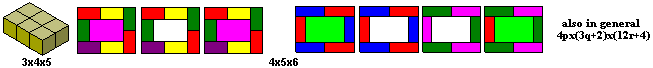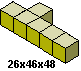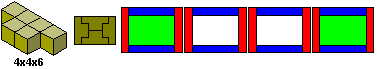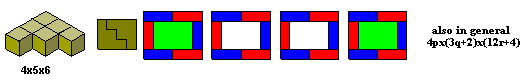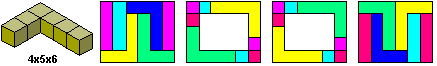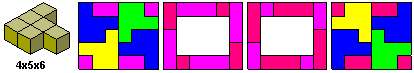Heptacubes

PHB boxes for heptacubes must of one of seven forms

 A: 7p x (7q+2) x r B: (7p+1) x (7q+3) x (7r+4) C: (7p+1) x (7q+5) x (7r+6) D: (7p+4) x (7q+6) x (7r+6) E: (7p+4) x (7q+4) x (7r+4) F: (7p+5) x (7q+5) x (7r+5) G: (7p+3) x (7q+3) x (7r+5)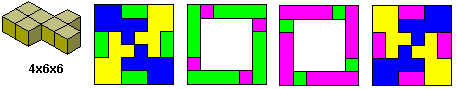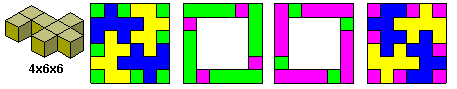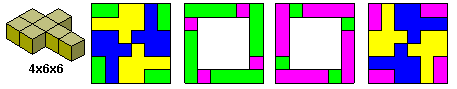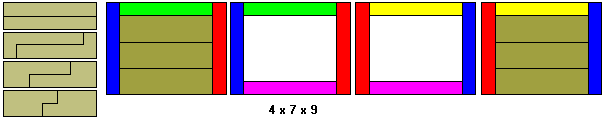The following information is from Patrick Hamlyn -

The minimum PHB has the same dimensions as the minimum box. It tiles an open box of size 4x6x6 in two symmetric ways, which gives four tilings of the 8x6x6 PHB (to see all solutions click on the image).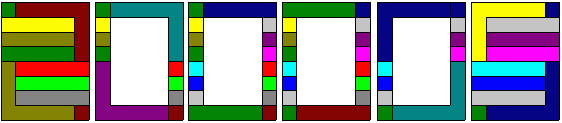Patrick Hamlyn has made a complete list of PHBs (allowing multiple boxes) for a full set of hexominos:

3x5x175x7x9 (solution by Mike Reid)3x3x5, 5x6x8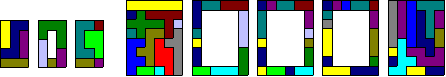3x5x5 3x4x143x5x5 4x6x83x3x11 3x6x83x5x7 3x6x8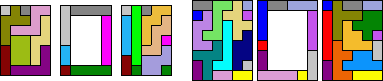4x5x6 3x5x93x3x5 3x4x5 3x3x14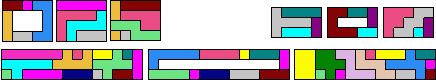3x3x5 3x4x5 3x4x11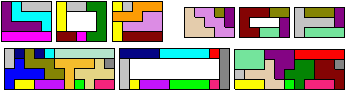3x3x5 3x4x5 5x5x6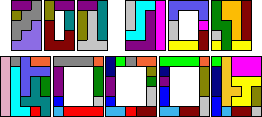3x3x5 3x3x8 3x5x83x3x5 3x4x8 3x4x8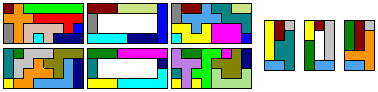3x4x5 3x3x8 3x3x11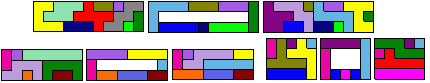3x4x5 3x3x8 3x5x73x3x8 3x5x5 3x5x63x3x5 3x3x5 3x3x5 3x4x8The tetrominoes, pentominoes and hexominoes can form 5 simultaneous PHBs: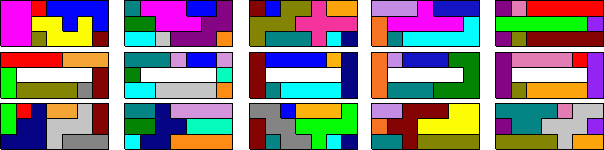Here are the trominos, tetrominos, pentominos and hexominos in a hollow cube, side 8